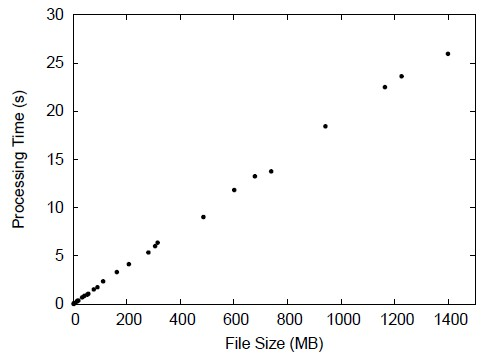In this project, we explored the performance of the popular hash function MD5 — Message Digest Algorithm Version 5. Firstly we introduce the algorithm in detail. Then we check the algorithm’s complexity in two aspects: computational and space complexity. Furthermore we check the security aspects of MD5: whether it is feasible to find collision it using brute force, birthday attack, and cryptanalysis. Available cryptanalysis methods are introduced so that we can find out why MD5 is not considered secure any more.

The computational complexity of MD5 can be seen from the following diagram:The bash script used to generate the above diagram is as follows:

```#!/bin/sh
# Date:   Nov. 28th, 2009
# Usage:  ./timemd5.sh Directory_Name

USAGE='Usage: ./timemd5.sh Directory_Name'

if [ \$# != 1 ]
then
echo \$USAGE
exit
fi

if [ -d \$1 ]
then
DICTORY=\$1
else
echo \$USAGE
exit
fi

# Create a statics file
DATE=`date +%Y%m%d%H%M%S%N`
STATICS="\$HOME/statics.\$DATE"
if [ ! -e "\$STATICS"]
then
touch "\$STATICS"
fi
cat /dev/null >\$STATICS

cd \$DICTORY

# For each file in the directory,
# compute its MD5 value with 'md5sum'.
# And record the file size and the time
# it takes to compute its MD5 hash.
find \$PWD -iname "*" |\
do
if [ -f "\$i" ]
then
echo "Calculating MD5 for: \$i"
SIZE=`du -m "\$i" |cut -f1`
(time -f "%e" -o /tmp/time md5sum \
"\$i")1> /dev/null
TIME=`cat /tmp/time`
echo "\$SIZE\t\t\$TIME" >>\$STATICS
fi
done

rm /tmp/time
echo "Size\t\tTime"
cat \$STATICS

# Draw a more straightforward graph
# using 'gnuplot'
cd \$HOME
GNUPLOTFILE="plot"
echo "set terminal postscript eps 22" \
>\$GNUPLOTFILE
echo "set output \"graph.eps\"" \
>>\$GNUPLOTFILE
echo "set key left top Left" \
>>\$GNUPLOTFILE
echo "setxlabel \"File Size (M)\"" \
>>\$GNUPLOTFILE
echo "set ylabel \"Processing Time (s)\"" \
>>\$GNUPLOTFILE
echo "plot [0:1500][0:50] \""\$STATICS"\" \
with points ps 1 pt 7 title \"\"" \
>>\$GNUPLOTFILE
gnuplot <\$GNUPLOTFILE

evince graph.eps&

#rm \$GNUPLOTFILE
#rm \$STATICS